PetroSkills Blog
By:

# Solubility of Gases in Water: Part 2

Part 2. Practical Applications

Why should we care about solubility of gases in water?

The solubility of hydrocarbons and non-hydrocarbons like carbon dioxide and hydrogen sulfide in water is of interest for oil and gas production and processing facilities, dealing with water treatment, and disposal facilities from an environmental aspect. Understanding the water solubility of gases in produced water is critical for piping and equipment sizing to ensure there is adequate vapor handling capacity, for example, in the production separators, gas compressors, vapor recovery units or possibly thermal oxidizers. This is important to ensure the facilities have adequate capacity to minimize hydrocarbon emissions. In addition, determination of the solubility of these components in aqueous phase is critical in the study of the gas hydrates kinetics .

In Part 1 of this series, January 2021 tip of the month (TOTM) , we focused on the solubility of light hydrocarbon gases such as CH4, C2H6, C3H8, iC4H10, and nC4H10­. We reviewed and presented example available experimental solubility data, example thermodynamic model. For that case study, we presented diagrams to show the effect of temperature and pressure on the solubility of these light hydrocarbon gases in water.

Case Study

To investigate the estimation of solubility of light hydrocarbon gases in water, we will consider two lean gas mixtures with the composition shown in Table 1.

Table 1. Compositions of two gas mixtures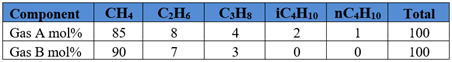In Part 1, for Gas A, we obtained the solubility of each component in water from ProMax  using the Peng-Robinson equation of state. Four isotherms of 5°C, 10°C, 20°C, and 30°C (41°F, 50°F, 68°F, and 86°F) were selected. For each isotherm, pressure was varied from 200 kPa to 8000 kPa (29 psia to 1160 psia). The calculated gas solubility results, std m3 of gas per m3 of water (SCF of gas per bbl of water) are presented in Figures 2 and 3 (See Appendix A).

In this tip, we will review and answer the following questions:

1. How do I convert the gas solubility from std m3 of gas per m3 of water (scf of gas per bbl of water) to mol fraction?

2. Is there a short cut method for estimating gas solubility in water and how can I use it?

3. How can I use the solubility charts of Figures 2 and 3 for compositions of Gas A to estimate solubility of another gas such as Gas B with different compositions?

4. How do I convert the gas solubility from mol fraction to std m3 of gas per m3 of water (scf of gas per bbl of water)?

Q1: Example 1 – Conversion of solubility units from volumetric rates to mol %

From Figure 3 of Part 1, for temperature of 30 °C (86 °F) and pressure of 6 MPa (870 psia) the solubility of gases in volumetric units are: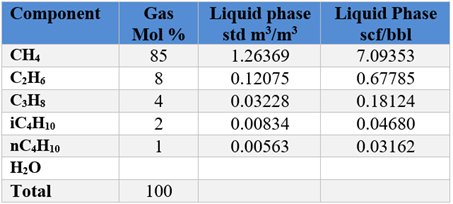Convert gas solubility units from volumetric rates to mol %. Density of water is 1000 kg/m3 (62.4 lbm/ ft3) and MW = 18

SI Solution:

Assume a basis of one m3 of liquid water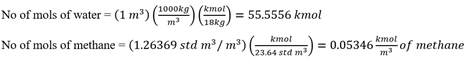Repeat the above calculation for each gas component and populate the second column from right of Table 2 SI. We also add 55.5556 kmol of water. The sum is 55.6161 kmol.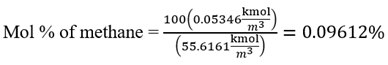Repeat the above calculation for each gas component and populate the last column of Table 2 SI.

Table 2 SI. Conversion of volumetric solubility to mol%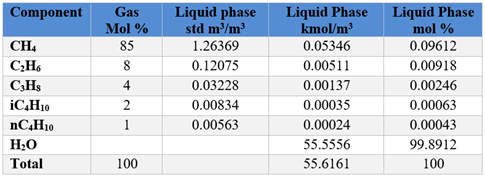FPS Solution:

Assume a basis of one bbl of liquid water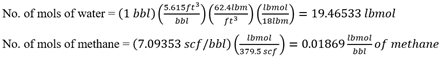Repeat the above calculation for each gas component and populate the second column from right of Table 2 FPS. We also add 19.4653 lbmol of water. The sum is 19.4865 lbmols.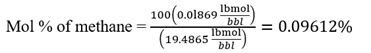Repeat the above calculation for each gas component and populate the last column of Table 2 FPS.

Table 2 FPS. Conversion of volumetric solubility units to mol%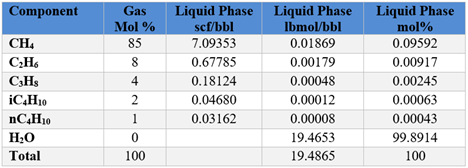Q2: Solubility of light Hydrocarbon Gases – Henry’s Law

Henry’s law is one of the methods for gas solubility when the concentration of components in the liquid phase is low and the assumption of ideal gas is valid at low pressure. Under these conditions Henry’s law can be written by Equation 1,

Pyi=Hixi                                                                                                                                           (1)       Where P, Hi, yi and xi are the pressure, Henry’s constant, and mol fraction in the vapor and liquid phases of component i, respectively. For a mixture at high pressure, the non-ideality of the gas phase is described by the vapor phase fugacity coefficient, ϕiV  in Equation 2.

PyiϕiV=Hixi                                                                                                                                      (2)

ϕiV should be calculated at prevailing conditions by an equation of state.

Henry’s constant of a component is experimentally determined. Also, it is assumed to be independent of its concentration but, this constant is a function of temperature. Figure 1 shows Henry’s constant for gaseous components of reservoir fluids in water .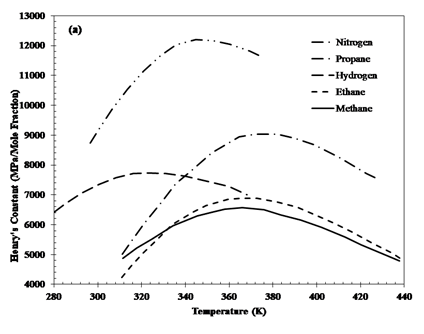Figure 1. Henry’s constant for different gases 

In addition, Henry’s constant dependency on pressure can be calculated using the following relation: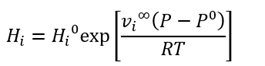(3)

Where Hi0  and vi  are Henry’s constant at P0 and the partial molar volume of component i in the solvent at infinite dilution. vi  is assumed to be constant over the prevailing composition and pressure ranges. Equation 3 is known as Krichevsky-Kazarnovsky equation. Average of partial molar volume for methane, ethane, propane and nitrogen are 0.040, 0.055, 0.080 and 0.035 m3/kmol (0.641, 0.881, 1.281, 0.561 ft3/lbmol), respectively .

Q2: Example 2

For the gas mixture A of Table 1, estimate the methane, ethane, and propane gas solubility in water using Henry’s law at the following conditions: T= 30 °C (86 °F) and P = 8 MPa (1160 psia)

Compare the results with the ProMax results reported in Part 1.

Solution: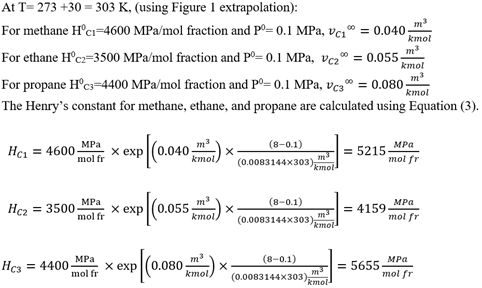The solubility of each gas component can be calculated using Equation (2).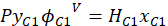(2)

Because of the low volatility of water in comparison to methane: yC1=0.85. Also, from Table 1A in Appendix A, the methane fugacity coefficient is ϕC1V=0.896. The gas solubility is as follows: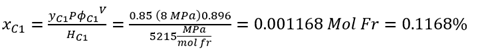Based on example 1, the ProMax value of solubility is 0.1223%.

From Table 1A in Appendix A, the ethane fugacity coefficient is ϕC2V=0.606.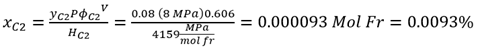Based on example 1, the ProMax value of solubility is 0.0104%.

From Table 1A in Appendix A, the propane fugacity coefficient is ϕC3V=0.442.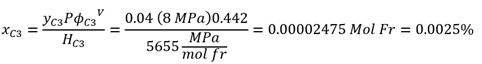Based on example 1, the ProMax value of solubility is 0.0025 %.

In this example, the accuracy of results has been improved by calculating the ϕiV using an equation of state.

Table 2. Example 2 Summary of results for GAS A and comparison with ProMax results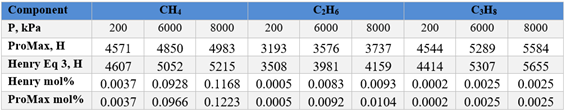Q3: Example 3

For the gas mixture B of Table 1, estimate the methane, ethane, and propane gas solubility in water at T= 30 °C (86 °F) and P= 7 MPa (1015 psia)

a. Using results of ProMax for gas mixture A at T= 30 °C (86 °F) and P= 8 MPa (1160 psia) listed in Table 2 and Equations 3 and 4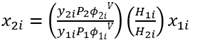(4)

Subscript 1 represents Gas A and its conditions and properties and subscript 2 represents Gas B and its conditions and properties.

b. Using Equations 2 and 3

c. Using of ProMax results for gas mixture B at T= 30 °C (86 °F) and P= 7 MPa (1015 psia) in Table 2A SI of Appendix A.

Solution

Method a:

From example 2, for gas mixture A at T= 30 °C (86 °F) and P1= 8 MPa (1160 psia)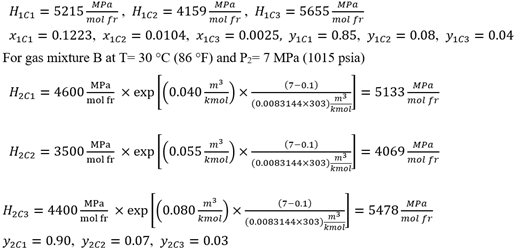Using Equation 4 and fugacity coefficients from Table 1A of Appendix A: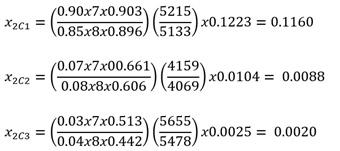Method b.

For gas mixture B at T= 30 °C (86 °F) and P2= 7 MPa (1015 psia) from Method a.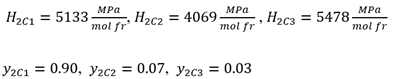Using Equation 2 and fugacity coefficients from Table 1A of Appendix A, the gas solubility for each component is as follows: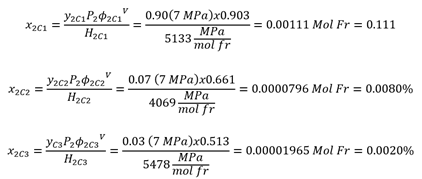Method c.

For gas mixture B at T= 30 °C (86 °F) and P2= 7 MPa (1015 psia) from Table 2A SI of Appendix A.

x2C1=0.1155  mol%,  x2C2=0.0089 mol%, x2C3=0.0020 mol%

Table 3. Example 3 Summary of results for GAS B and comparison with ProMax results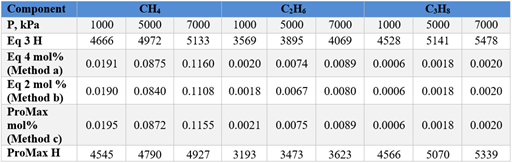Q4: Example 4 – Conversion of solubility units from mol % to volumetric rates

From Appendix A (also Example 3), for temperature of 30 °C (86 °F) and pressure of 7 MPa (1015 psia) the solubility of gases in mole % are: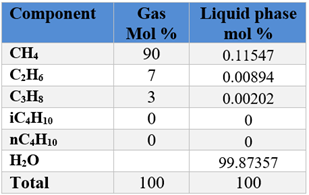Convert the solubility units from mol % to volumetric rates. Density of water is 1000 kg/m3 (62.4 lbm/ ft3) and MW = 18

SI Solution:

Assume a basis of one m3 of liquid water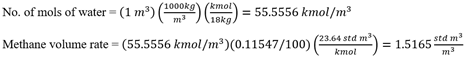Repeat the above calculation for each gas component and populate the second column from the right of Table 4.

Table 4 SI. Conversion of mol % solubility to volumetric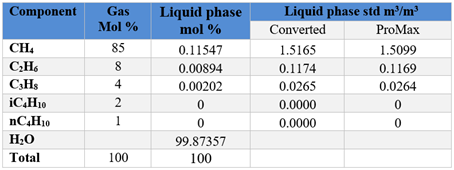FPS Solution:

Assume a basis of one bbl of liquid water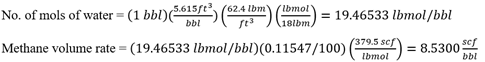Repeat the above calculation for each gas component and populate the second column from right of Table 4.

Table 4 FPS. Conversion of mol % solubility to volumetric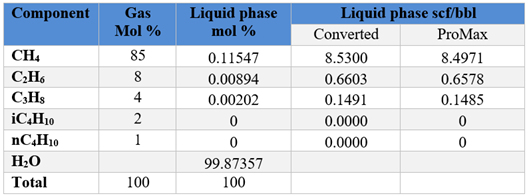Summary

This TOTM presented basic thermodynamic models and estimation methods for the solubility of light hydrocarbon gases, i.e., methane through normal butane, in water as a function of pressure, temperature, and composition. By incorporating the composition dependent fugacity coefficients predicted by the SRK EOS, at high pressures the accuracy of results improved considerably. The tip demonstrated the use of solubility charts of Figures 2 and 3 (Table 2A) for compositions of Gas A to estimate solubility of another gas such as Gas B with different compositions.

To learn more about similar cases and how to minimize operational problems, we suggest attending our G4 (Gas Conditioning and Processing), G5 (Practical Computer Simulation, and Applications in Gas Processing) courses.

By: Mahmood Moshfeghian, Ph.D.References:

1. Sloan, E.D., Koh, C.A., Clathrate Hydrates of Natural Gases, 3rd ed., CRC Press, 2008.

2. Moshfeghian, M., https://petroskills.com/blog/entry/00_totm/jan21-fac-Solubility-of-Gases-in-WaterP1, Light Hydrocarbon gases, Jan 2021

3. ProMax 5.0, Build 5.0.20034.0, Bryan Research and Engineering, Inc., Bryan, Texas, 2020.

4. A. Danesh, PVT and phase behaviour of petroleum reservoir fluids. Elsevier, 1998.

Appendix A

Figure 2. Variation of solubility of CH4, C2H6, C3H8, iC4H10, and nC4H10 with pressure and at low temperatures

Figure 3. Variation of solubility of CH4, C2H6, C3H8, iC4H10, and nC4H10 with pressure and at moderate temperatures

Table 1A. Fugacity coefficient for gas mixtures A and B at 30°C (86°F) predicted by the SRK EOS

Table 2A (SI). Solubility data for gas mixtures A and B calculated by ProMax 

Table 2A (FPS). Solubility data for gas mixtures A and B calculated by ProMax 

## Sidebar

### Featured posts###### Hydrogen Series Part 2: Hydrogen compression and transportation by transmission pipeline – What are permissible conditions and limitations and their associated challenges?
by Kindra Snow-McGregor

### Popular tags

#Renewables #LNG #Unconventionals